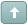Error

 Previous Topic Next Topic
 aksh #1 Posted : Wednesday, March 13, 2013 5:06:25 AM(UTC)Rank: MemberGroups: Registered, Registered UsersJoined: 1/20/2007(UTC)Posts: 15Was thanked: 1 time(s) in 1 post(s) I had actually posted this a few years ago but nobody commented on it.I plotted a 20-period Modified Moving Average by Joe Sharp with the formula given below and then plotted MetaStock's 20-period Linear Regression indicator [ LinearReg(C,20) ] on top of it and found that they plot on top of each other and the end values on the title bar are same. Then I did the same with a 10-period MMA and a 10-period Linear Regression Indicator and they also plotted alike.Therefore, both are actually one indicator with different names.{Modified Moving Average - TASC Jan 2000 by Joe Sharp} n:=20;tn:=Mov(C,n,S);s1:=((n-1)/2)*C + ((n-3)/2)*Ref(C,-1) + ((n-5)/2)*Ref(C,-2)+ ((n-7)/2)*Ref(C,-3) + ((n-9)/2)*Ref(C,-4)+ ((n-11)/2)*Ref(C,-5) + ((n-13)/2)*Ref(C,-6)+ ((n-15)/2)*Ref(C,-7) + ((n-17)/2)*Ref(C,-8)+ ((n-19)/2)*Ref(C,-9) + ((n-21)/2)*Ref(C,-10)+ ((n-23)/2)*Ref(C,-11) + ((n-25)/2)*Ref(C,-12)+ ((n-27)/2)*Ref(C,-13) + ((n-29)/2)*Ref(C,-14)+ ((n-31)/2)*Ref(C,-15) + ((n-33)/2)*Ref(C,-16)+ ((n-35)/2)*Ref(C,-17) + ((n-37)/2)*Ref(C,-18)+ ((n-39)/2)*Ref(C,-19);tn+(6*s1)/((n+1)*n);So, why go through the hassle of plotting the above code for a 20-period MMA when it can be easily plotted as LinearReg(C,20).Any comments?ArunWanna join the discussion?! Login to your Discussions forum accountor Register a new forum account.Users browsing this topic
Forum Jump
You cannot post new topics in this forum.
You cannot reply to topics in this forum.
You cannot delete your posts in this forum.
You cannot edit your posts in this forum.
You cannot create polls in this forum.
You cannot vote in polls in this forum.